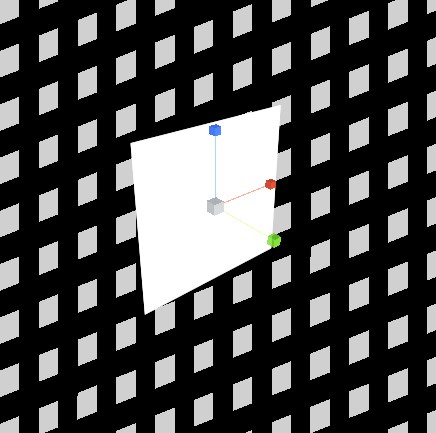# post shader: reconstruct worldspace plane coordinates

i want to create a postprocess-shader, which reconstructs the xy-coordinates on a plane at z=0, to then use it further.

somehow, i am not able to properly reconstruct the world positions from the Screenspace coordinates.

_

my fragment shader looks like this:

``````                fixed4 col;
float3 rayStart = mul(unity_CameraToWorld, float4(i.uv, .0,0));
float3 rayEnd   = mul(unity_CameraToWorld, float4(i.uv, 1,0));

float3 direction = rayEnd - rayStart;

float distanceToDrawPlane = (.0 - rayStart.z) / direction.z;
float3 position = rayStart + direction * distanceToDrawPlane;

col.xyz = position;

//debug grid pattern
col.xyz = frac(position.x*10) < .5;
col.xyz *= frac(position.y * 10) < .5;

return col;
``````

while the grid is on the correct axis, it has some problems:

• it moves with the camera, instead of keeping its position relative to the origin
• the perspective is always orthographic, even in the perspective camera.

now, i tried different approaches, but was unable to make it work properly. other code ive found uses “ComputeViewSpacePosition”, but i couldnt find where this function comes from.

does anyone know how to do this conversion properly, or has any example in mind?It’s hard to tell exactly what your code is doing without also seeing what ‘i.uv’ consists of. That said, here’s how I do it;

To start off, we’re gonna have to pass some info to the shader - as much as Unity does define the camera matrices, from my experience the view-space matrices are never correct so it’s easier to just do it ourselves.

``````m_Material.SetMatrix ("_ViewToWorld", m_Camera.cameraToWorldMatrix);
m_Material.SetMatrix ("_InvProjectionMatrix", m_Camera.projectionMatrix.inverse);
``````

We also need to ‘undo’ the camera projection for this to work (and not appear orthographic as you said), so pass in the inverse projection matrix as well.

When it comes to the shader, the basic process is as follows:

In the vertex shader, we need to calculate the camera frustum corners that lie on the far clip plane, which we can do using the input UV coordinates and the inverse projection matrix.

``````float4x4 _InvProjectionMatrix;
float4x4 _ViewToWorld;

struct appdata
{
float4 vertex : POSITION;
float2 uv : TEXCOORD0;
};

struct v2f
{
float4 pos : SV_POSITION;
float2 uv : TEXCOORD0;
float4 viewDir : TEXCOORD1;
};

v2f vert (appdata v)
{
v2f o;
o.pos = UnityObjectToClipPos (v.vertex);
o.uv = v.uv;
// Input position is just the UVs going from [-1,1] at the far clip plane (1.0 because we manually passed in the matrix, which Unity stores via GL convention)
o.viewDir = mul (_InvProjectionMatrix, float4 (o.uv * 2.0 - 1.0, 1.0, 1.0));
return o;
}
``````

Then, in the fragment shader, we can take those points on the far clip plane and convert them into world space using the inverse view matrix we passed in earlier. If you wanted to reconstruct the position of actual in game objects you could also multiply by scene depth at this point.

``````// Perspective correction
float3 viewPos = i.viewDir.xyz / i.viewDir.w;
// Normalize for view-space view dir
float3 viewDir = viewPos / abs (viewPos.z);

// If you need normalized world-space view direction use this (don't normalize() otherwise you'll get perspective distortion)
float3 worldSpaceViewDir = mul ((float3x3)_ViewToWorld, viewDir);

// Start at the camera's position
float3 rayStart = _WorldSpaceCameraPos.xyz;
// Convert point on the far clip plane to world-space
float3 rayEnd = mul (_ViewToWorld, float4 (viewPos, 1.0));
``````